# Generics in Java

## Generics in Java with Examples

In this article, I am going to discuss Generics in Java with Examples. Please read our previous article where we discussed Sorting Collections in Java with Examples. As part of this article, we are going to discuss the following pointers in detail.

1. Generics in Java
2. Advantages of Generics in Java
3. Generic Class, Interfaces and Methods in Java
4. Generic Programming in Java
5. The wildcard in Java Generics
6. Types of Wildcards in Java
7. Upper Bounded Wildcards in Java
8. Unbounded Wildcards in Java generics
9. Lower Bounded Wildcards in Java
##### Generics in Java:

The Generics concept is introduced in Java 1.5 Version which is used to achieve generic programming and resolving the problems of type safety and need for typecasting. Generics can also be called as generic parameter types.

The Generics concept can be used only for storing objects but not for primitive values. The Generics can be called type erasers because the generic information is available only up to compilation, once compilation is done then all the generic information will be erased.

Using the generics concept we can achieve compile-time polymorphism. This generic concept looks like a template concept in C++. We can apply the generics concept for classes, interfaces, and for methods.

##### Advantages of Generics in Java
1. We can write a method/class/interface once and use it for any type we want.
2. We can hold only a single type of object in generics. It doesn’t allow to store other objects.
3. Individual Type Casting is not needed.
4. By using generics, we can implement algorithms that work on different types of objects and at the same, they are type-safe too.
5. It is checked at compile time so the problem will not occur at runtime. The good programming strategy says it is far better to handle the problem at compile time than runtime.
##### Generic Class in Java:

A generic class is a class that can hold any kind of object. To create a Generic class we have to specify generic type <T> after the class name. The syntax is given below.Note: We can specify n number of generic types with a class like follows:

class ClassName<T1, T2, T3,…..>{
}

##### Example to understand Generic Class in Java:
```class MyGen < T >
{
T obj;

{
this.obj = obj;
}

T get ()
{
return obj;
}
}

class GenericClassDemo
{
public static void main (String args[])
{
MyGen < Integer > m = new MyGen < Integer > ();
System.out.println (m.get ());
}
}```

Output: 2

##### Generic Interfaces in Java

We can also create a generic interface by specifying the <T> after the interface name. The syntax is given below.##### Example to Understand Generic Interfaces in Java:
```interface Tester < T >
{
void show (T o);
}

//Implementing the generic interface without specifying any generic parameter
class Imp1 implements Tester
{
public void show (Object o)
{
System.out.println(o);
}
}

//Implementing the generic interface by specifying generic parameter as Integer
class Imp2 implements Tester < Integer >
{
public void show (Integer o)
{
System.out.println(o);
}
}

//Implementing the generic interface by specifying generic parameter as String
class Imp3 implements Tester < String >
{
public void show (String o)
{
System.out.println(o);
}
}

//Implementing  the generic interface whose implementation class is also a generic class
class Imp4 < T > implements Tester < T >
{
public void show (T o)
{
System.out.println(o);
}
}

public class GenericInterface
{
public static void main (String[]args)
{
Imp1 i1 = new Imp1 ();
i1.show (10);
i1.show ("abc");
i1.show (true);

Imp2 i2 = new Imp2 ();
i2.show (10);
// i2.show("abc"); - Invalid

Imp3 i3 = new Imp3 ();
i3.show ("abc");
// i3.show(10.0); - Invalid

Imp4 < Integer > i4 = new Imp4 < Integer > ();
i4.show (10);
}
}```
###### Output: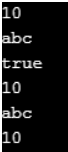##### Generic Method in Java:

A generic method is a method that can take any kind of parameter. To create the generic method we have to specify the generic type <T> before the return of the method. The syntax to use a generic method is given below.##### Example to Understand Generic Methods in Java:
```class Demo
{
<T> void show (T o)
{
System.out.println ("o=" + o);
}
}

public class GenericMethods
{
public static void main (String[]args)
{
Demo d = new Demo ();
d.show (10);
d.show (10.0);
d.show ("abc");
d.show ('c');
d.show (true);
d.show (10f);
d.show (10L);
}
}```
###### Output: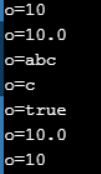##### Generic Programming in Java

Generic programming means reusing the same code for storing different types of objects.

##### Example: Java Program without a generic programming approach
```class Demo1
{
Integer e;
{
this.e = e;
}
Integer getValue ()
{
return e;
}
}

public class GPTest1
{
public static void main (String[]args)
{
Demo1 d = new Demo1 ();
Integer i = d.getValue ();
System.out.println ("i=" + i);
}
}```

Output: i = 10

In the above program, class Demo1 can hold only Integer objects but it cannot hold any other objects like Float objects, String objects, etc. It means we have type safety for the data and while retrieving typecasting is optional but there is no generic programming approach.

To resolve this problem we have to create many classes to store the different objects which will increase the code size and maintenance becomes complicated. To achieve generic programming in our application we have to use Java’s super most class called Object (up to Java 1.5).

##### Example: Program using object
```class Demo2
{
Object e;
{
this.e = e;
}
Object getValue ()
{
return e;
}
}

public class GPTest2
{
public static void main (String[]args)
{
Demo2 d = new Demo2 ();
Integer i = (Integer) d.getValue ();
System.out.println ("i=" + i);
String str = (String) d.getValue ();
System.out.println ("str=" + str);
Double dd = (Double) d.getValue ();
System.out.println ("dd=" + dd);
}
}```

Output: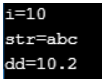Note: In the above program class Demo2 can hold any kind of object like Integer Objects, Float Objects, String Objects, etc. It means here we have a generic programming approach.

##### But in this approach we have the following two drawbacks:
1. There is no type safety for the data, for example, if we want to store salary values we should enter all the integer values and it will accept but in the middle unexpectedly we enter any wrong value like String or double or other objects still it will accept so that there is no type safety.
2. While retrieving the data, typecasting is mandatory.

To achieve generic programming and resolving the problems of no type of safety and need for typecasting, Java people have introduced a concept called generics.

##### Example: Program with Type Safety using Generics
```class Demo3 <T>
{
T e;
{
this.e = e;
}
T getValue ()
{
return e;
}
}

public class GPTest3
{
public static void main (String[]args)
{
// case-1
Demo3 < Integer > d1 = new Demo3 < Integer > ();
Integer i = d1.getValue ();
System.out.println ("i=" + i);

//case-2
Demo3 < String > d2 = new Demo3 < String > ();
String str = d2.getValue ();
System.out.println ("str=" + str);

//case-3
Demo3 d3 = new Demo3 ();
Integer i1 = (Integer) d3.getValue ();
System.out.println ("i1" + i1);
String str1 = (String) d3.getValue ();
System.out.println ("str1=" + str1);
}
}```
###### Output: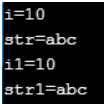##### Note:
1. In the above program, class Demo13 can store any kind of object which means here we have a generic programming approach.
2. In case-1 object, d1 is created by specifying generic parameter as Integer so object d1 can hold only Integer objects (type safety) and while retrieving the data type casting is optional.
3. In case-2 object, d2 is created by specifying generic parameter as String so object d2 can hold only String objects (type safety) and while retrieving the data type casting is optional.
4. But in case-3 object d3 is created without specifying any generic parameter type, so object d3 can hold any kind of objects (no type safety) and while retrieving the data type casting is mandatory.
##### The wildcard in Java Generics

In generic code, the question mark (?), called the wildcard, represents an unknown type. The wildcard can be used in a variety of situations: as the type of a parameter, field, or local variable; sometimes as a return type (though it is better programming practice to be more specific). The wildcard is never used as a type argument for a generic method invocation, a generic class instance creation, or a supertype.

##### Example to understand WildCard in Generics
```import java.util.*;
abstract class Shape
{
abstract void draw ();
}
class Rectangle extends Shape
{
void draw ()
{
System.out.println ("drawing rectangle");
}
}
class Circle extends Shape
{
void draw ()
{
System.out.println ("drawing circle");
}
}
class WildcardDemo
{
//creating a method that accepts only child class of Shape
public static void drawShapes (List < ? extends Shape > lists)
{
for (Shape s : lists)
{
s.draw ();		//calling method of Shape class by child class instance
}
}
public static void main (String args[])
{
List < Rectangle > list1 = new ArrayList < Rectangle > ();

List < Circle > list2 = new ArrayList < Circle > ();

drawShapes (list1);
drawShapes (list2);
}
}```

Output: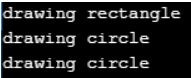#### Types of Wildcards in Java

##### Upper Bounded Wildcards in Java

You can use an upper bounded wildcard to relax the restrictions on a variable. For example, say you want to write a method that works on List<Integer>, List<Double>, and List<Number>; you can achieve this by using an upper bounded wildcard.

To declare an upper-bounded wildcard, use the wildcard character (‘?’), followed by the extends keyword, followed by its upper bound. Note that, in this context, extends is used in a general sense to mean either “extends” (as in classes) or “implements” (as in interfaces).

Syntax : public static void process(List<? extends Foo> list){…..}

##### Implementation:
```public static double sum(List<Number> list){
double sum = 0;
for(Number n : list){
sum += n.doubleValue();
}
return sum;
}```
##### Example to Understand Upper Bounded Wildcards in Java
```import java.util.ArrayList;
import java.util.List;
public class UpperBoundDemo
{
public static void main (String[]args)
{
List < Integer > intl = new ArrayList <> ();
double sum = sum (intl);
System.out.println ("Total Sum = " + sum);
}
public static double sum (List < ? extends Number > list)
{
double sum = 0;
for (Number n:list)
{
sum += n.doubleValue ();
}
return sum;
}
}```

Output: Total Sum = 49.0

##### Unbounded Wildcards in Java generics

The unbounded wildcard type is specified using the wildcard character (?), for example, List<?>. This is called a list of unknown type. There are two scenarios where an unbounded wildcard is a useful approach: If you are writing a method that can be implemented using functionality provided in the Object class.

When the code is using methods in the generic class that doesn’t depend on the type parameter. For example, List.size or List.clear. In fact, Class<?> is so often used because most of the methods in Class<T> do not depend on T.

##### Implementation:
```public static void printData(List<?> list){
for(Object obj : list){
System.out.print(obj + "::");
}
}```
##### Example to understand UnBounded Wildcards in Java generics
```import java.util.ArrayList;
import java.util.List;
import java.util.Arrays;
class UnboundedDemo
{
public static void main (String[]args)
{
List < Integer > integerList = Arrays.asList (6, 3, 10, 7);
print (integerList);
System.out.println ("\n----------");
List < String > stringList = new ArrayList < String > ();
print (stringList);
}
public static void print (List < ? >list)
{
for (Object input : list)
{
System.out.print (input + " ");
}
}
}```
###### Output: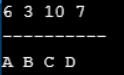##### Lower Bounded Wildcards in Java

The Upper Bounded Wildcards section shows that an upper bounded wildcard restricts the unknown type to be a specific type or a subtype of that type and is represented using the extends keyword. In a similar way, a lower bounded wildcard restricts the unknown type to be a specific type or a supertype of that type.

Syntax : Collectiontype <? super A>

##### Implementation:
```public static void addIntegers(List<? super Integer> list){
}```
##### Example to understand Lower Bounded Wildcards in Java
```import java.util.ArrayList;
import java.util.List;
class LowerBoundDemo
{
public static void main (String[]args)
{
List < Object > list = new ArrayList < Object > ();

for (Object value:list)
{
System.out.print (value + " ");
}
}

public static void addIntegers (List < ? super Integer > list)
{
System.out.println (list);
}
}
```

Output: 10 23 3

In the next article, I am going to discuss Multi-threading in Java with examples. Here, in this article, I try to explain Generics in Java with Examples and I hope you enjoy these Generics in Java with Examples article.